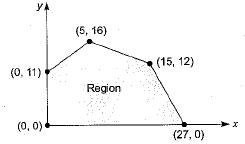Courses

# Test: Linear Programming - 1

## 10 Questions MCQ Test Topicwise Question Bank for Mechanical Engineering | Test: Linear Programming - 1

Description
This mock test of Test: Linear Programming - 1 for Mechanical Engineering helps you for every Mechanical Engineering entrance exam. This contains 10 Multiple Choice Questions for Mechanical Engineering Test: Linear Programming - 1 (mcq) to study with solutions a complete question bank. The solved questions answers in this Test: Linear Programming - 1 quiz give you a good mix of easy questions and tough questions. Mechanical Engineering students definitely take this Test: Linear Programming - 1 exercise for a better result in the exam. You can find other Test: Linear Programming - 1 extra questions, long questions & short questions for Mechanical Engineering on EduRev as well by searching above.
QUESTION: 1

Solution:
QUESTION: 2

Solution:
QUESTION: 3

### Shadow price in linear programming refers to the

Solution:
QUESTION: 4

The linear programming is used for optimization problems which satisfy the following conditions:
1. Objective function is expressed as a linear function of variables.
2. Resources are unlimited.
3. The decision variables are interrelated and non-negative.

Which of these statements is/are correct?

Solution:

LP is used for optimization problems having limited resource. Unlimited resources have nothing to do with optimization.

QUESTION: 5

In case of solution of a two variable linear programming problems by graphical method, one constraint line comes parallel to the objective function line. Then the problem will have

Solution:
QUESTION: 6

Find the maximum value of the objective function z = 4x + 6y where x ≥ 0 and y ≥ 0, subject to the constraints
- x + y ≤ 11
x + y ≤ 27
2x + 5y ≤ 90

Solution:At (0,0): z = 4(0) + 6(0) = 0
At (0, 11): z = 4(0)+ 6(11) = 66
At (5, 16): z = 4(5)+ 6(16) = 116
At (15, 12): z = 4(15) + 6(12) = 132
At (27,0): z = 4(27)+ 6(0) = 108

QUESTION: 7

The while solving a LP model graphically, the area bounded by the constraints is called

Solution:
QUESTION: 8

While solving a LP problem, infeasibility may be removed by

Solution:
QUESTION: 9

Which one of the following is true in case of simplex method of linear programming?

Solution:
QUESTION: 10

A feasible solution to an LP problem

Solution: